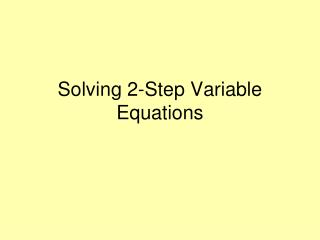# Solving 2-Step Variable Equations - PowerPoint PPT PresentationDownload PresentationSolving 2-Step Variable Equations

Solving 2-Step Variable EquationsDownload Presentation## Solving 2-Step Variable Equations

- - - - - - - - - - - - - - - - - - - - - - - - - - - E N D - - - - - - - - - - - - - - - - - - - - - - - - - - -
##### Presentation Transcript

1. Solving 2-Step Variable Equations

2. Two Step Equations Essential Question How are inverse operations used to solve two step equations? Why does order matter when solving two step equations?

3. What??? I just learned 1-step! Relax. You’ll use what you already know to solve 2-step equations.

4. Solving Two-Step Equations To find the solution to an equation we must isolate the variable. In other words, get the variable on one side of the equation by itself. We isolate the variable by performing operations that will eliminate (cancel) the other numbers from the expression.

5. Solving Two-Step Equations We have seen how to eliminate a constant (Addition Property of Equality) & how to eliminate a coefficient (Multiplication Property of Equality). What if an equation has both a constant and a coefficient to be eliminated? This is called a Two-Step Equation.

6. So, what’s first? You need to break up the equation into its two steps.

7. STEP 1 The first step will always be either adding or subtracting. (Think: Order of Operations backwards) + -

8. STEP 2 The second step will always be either multiplying or dividing. ÷ •

9. Let’s try a problem. 8x + 5 = 61 - 5 - 5 This problem has addition, so we need to subtract first. Whatever we do on one side, we have to do on the other. = 56 8x

10. Now, Step 2. 8x = 56 Whatever we do on one side, we have to do on the other. This problem has multiplication, so we need to divide now. 8 8 x 7 =

11. Let’s try another problem. 3a - 8 = 4 + 8 + 8 This problem has subtraction, so we need to add first. Whatever we do on one side, we have to do on the other. = 12 3a

12. Now, Step 2. 3a = 12 Whatever we do on one side, we have to do on the other. This problem has multiplication, so we need to divide now. 3 3 a 4 =

13. Let’s try another problem. z + 2 = 26 6 Whatever we do on one side, we have to do on the other. This problem has addition, so we need to subtract first. - 2 - 2 z 6 = 24

14. Now, Step 2. z = 24 6 6 • • 6 Whatever we do on one side, we have to do on the other. This problem has division, so we need to multiply now. z 144 =

15. Let’s try one more problem. m - 10 = 15 5 Whatever we do on one side, we have to do on the other. This problem has subtraction, so we need to add first. +10 +10 m 5 = 25

16. Now, Step 2. • 5 m = 25 5 5 • Whatever we do on one side, we have to do on the other. This problem has division, so we need to multiply now. m 125 =

17. Trick Question Does order matter when solving two step equations? Why or Why not? Order does matter because if you do any other math operation other than addition or subtraction first, you will end up with an incorrect solution